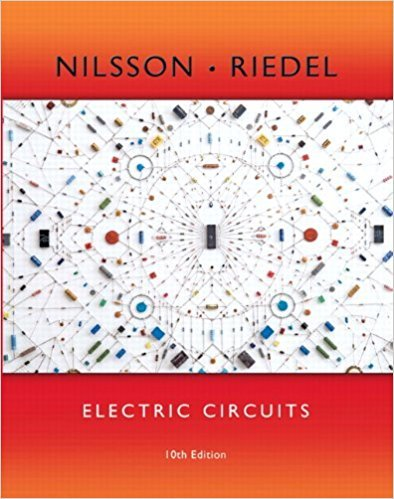×
×

# Show that the maximum value of the instantaneous power given by Eq. 10.9 is and that theISBN: 9780133760033 390

## Solution for problem 10.3 Chapter 10

Electric Circuits | 10th Edition

• Textbook Solutions
• 2901 Step-by-step solutions solved by professors and subject experts
• Get 24/7 help from StudySoup virtual teaching assistantsElectric Circuits | 10th Edition

4 5 1 286 Reviews
10
2
Problem 10.3

Show that the maximum value of the instantaneous power given by Eq. 10.9 is and that the minimum value

Step-by-Step Solution:
Step 1 of 3

BE 1500 – Fall 2016 HW 5 Due: October 13, 2016 (1:30 P.M.) 1. Write a code for repmat; the only built-in commands you are allowed to use are input, size, and disp. A=input('matrix '); [m,n]=size(A); z=input('replicated rows '); y=input('replicated columns '); for i=1:m for j=1:n B(i:m:z*m,j:n:y*n)=A(i,j);...

Step 2 of 3

Step 3 of 3

##### ISBN: 9780133760033

Unlock Textbook Solution